# The average age of a vehicle registered in the United States is 8 years (96 months)....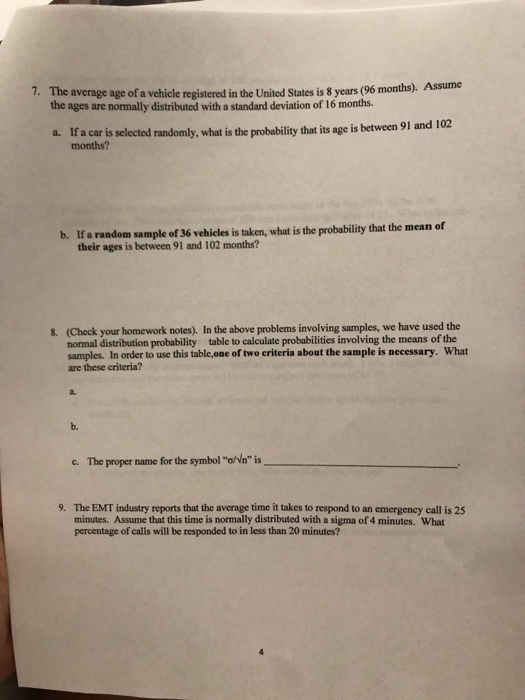The average age of a vehicle registered in the United States is 8 years (96 months). the ages are normally distributed with a standard deviation of 16 months. Assume 7. a car is selected randomly, what is the probabilty that its age is between 91 and 102 months? b. If a random sample of 36 vehicles is taken, what is the probability that the mean of their ages is between 91 and 102 months? 8. (Check your homework notes). In the above problems involving samples, we have used the table to calculate probabilities involving the means of the normal distribution probability samples. In order to use this table,one of two criteria about the sample is necessary. are these criteria? What a. b. c. The proper name for the symbol "a/Nn" is 9. The EMT industry reports that the average time it takes to respond to an emergency call is 25 minutes. Assume that this time is normally distributed with a sigma of 4 minutes. What percentage of calls will be responded to in less than 20 minutes?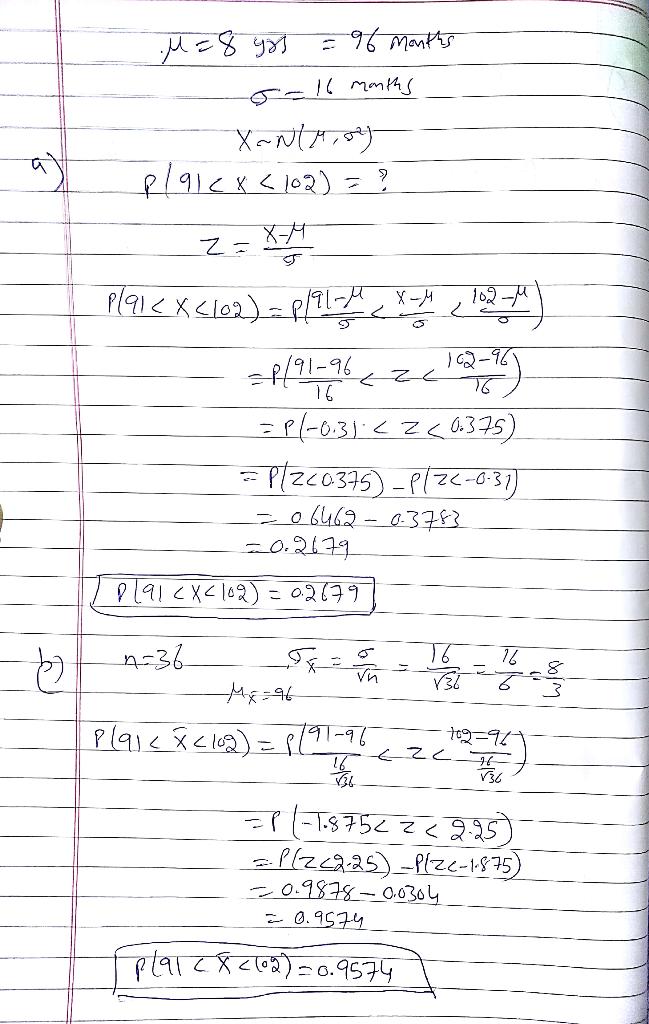#### Earn Coin

Coins can be redeemed for fabulous gifts.

Similar Homework Help Questions
• ### suppose the average age for registered voters is mu = 39.60 years with sigma = 11.60...

suppose the average age for registered voters is mu = 39.60 years with sigma = 11.60 years, and that the distribution of ages is normal. During a recent jury trial, a statistician noticed that the average age of the 12 jurors was 50.34 years, a) how likely is it to obtain a jury this old or older by chance? b)is it reasonable to conclude that this jury is not a random sample of registered voters? explain your answer. (a yes...

• ### Suppose the age that children learn to walk is normally distributed with mean 11 months and...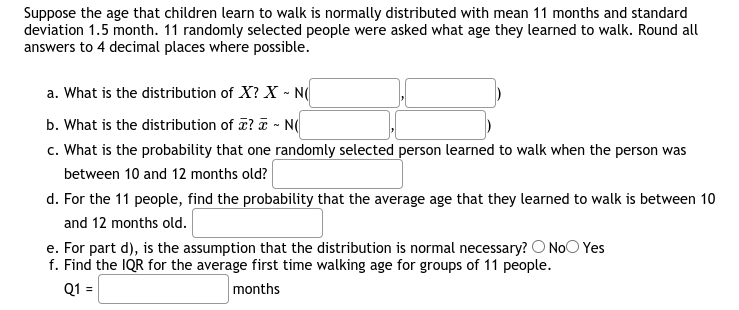Suppose the age that children learn to walk is normally distributed with mean 11 months and standard deviation 1.5 month. 11 randomly selected people were asked what age they learned to walk. Round all answers to 4 decimal places where possible. a. What is the distribution of X? X-NG b. What is the distribution of ? - N c. What is the probability that one randomly selected person learned to walk when the person was between 10 and 12 months...

• ### Ages of Proofreaders At a large publishing company, the mean age of proofreaders is 36.2 years...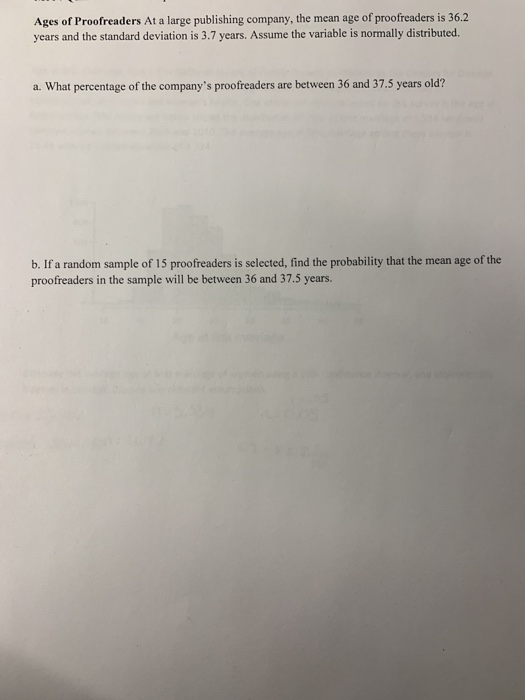Ages of Proofreaders At a large publishing company, the mean age of proofreaders is 36.2 years and the standard deviation is 3.7 years. Assume the variable is normally distributed. a. What percentage of the company's proofreaders are between 36 and 37.5 years old? b. If a random sample of 15 proofreaders is selected, find the probability that the mean age of the proofreaders in the sample will be between 36 and 37.5 years.

• ### Mayr et al. (1994) took an SRS of 240 children who visited their pediatric outpatient clinic. They found the following frequency distribution for the age (in months) of free (unassisted) walking amon...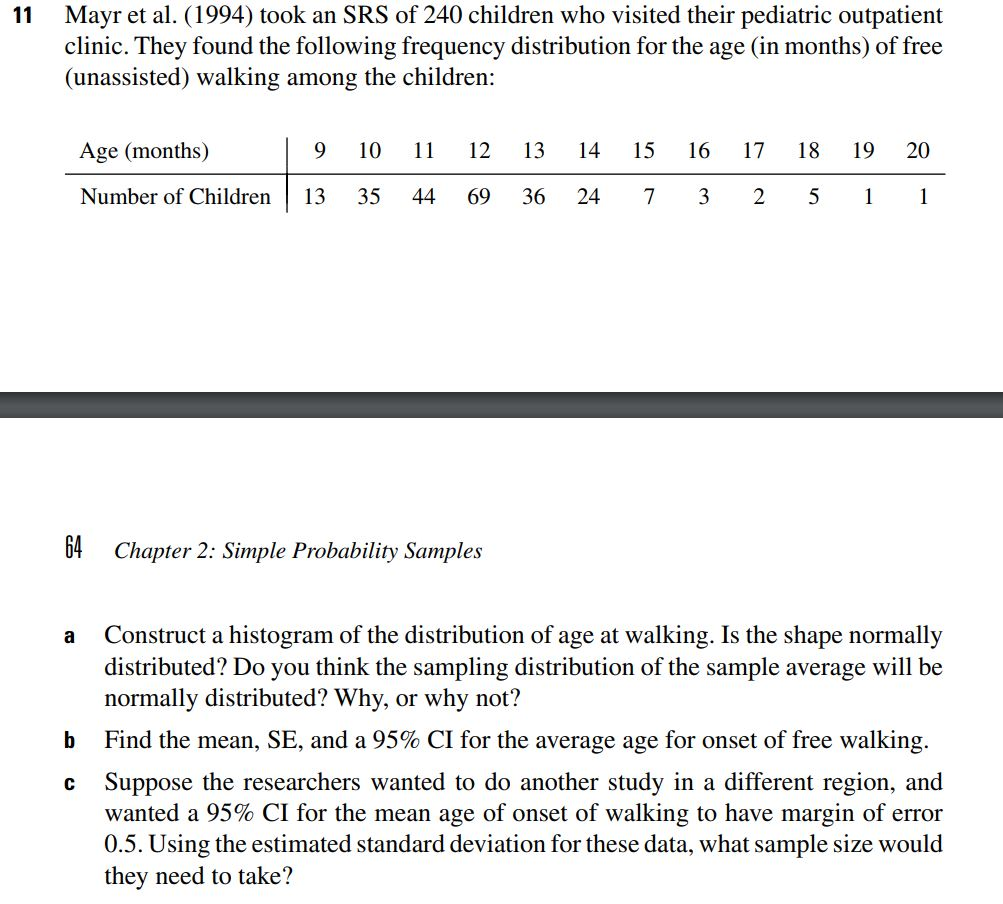Mayr et al. (1994) took an SRS of 240 children who visited their pediatric outpatient clinic. They found the following frequency distribution for the age (in months) of free (unassisted) walking among the children: 11 9 10 12 13 14 15 16 17 18 19 20 Age (months) Number of Children 13 35 4469 36 24 7 3251 64 Chapter 2: Simple Probability Samples Construct a histogram of the distribution of age at walking. Is the shape normally distributed? Do...

• ### Suppose that the age that children learn to walk is normally distributed with mean 12 months...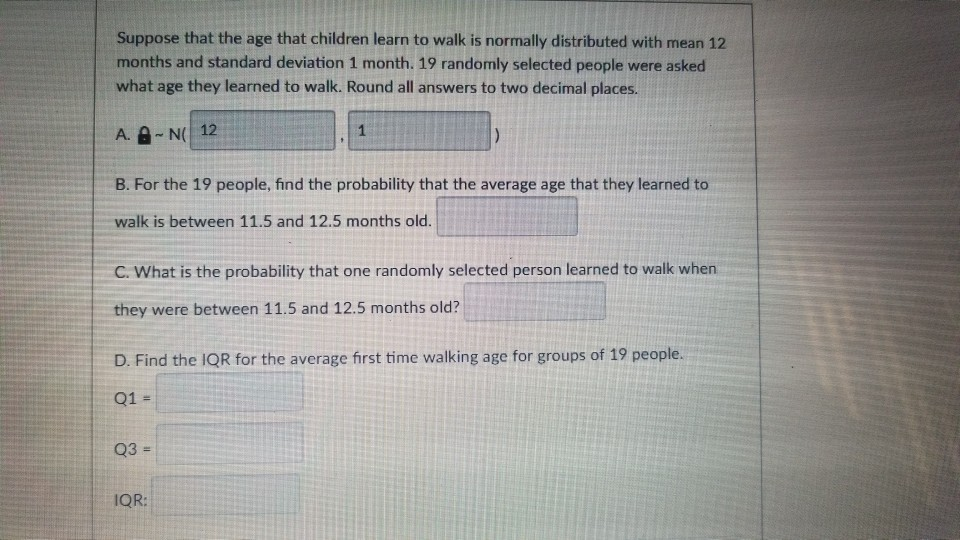Suppose that the age that children learn to walk is normally distributed with mean 12 months and standard deviation 1 month. 19 randomly selected people were asked what age they learned to walk. Round all answers to two decimal places. A. N 12 B. For the 19 people, find the probability that the average age that they learned to walk is between 11.5 and 12.5 months old C. What is the probability that one randomly selected person learned to walk...

• ### Suppose the age that children learn to walk is normally distributed with mean 11 months and...

Suppose the age that children learn to walk is normally distributed with mean 11 months and standard deviation 2.1 month. 33 randomly selected people were asked what age they learned to walk. Round all answers to 4 decimal places where possible. What is the distribution of X ? X ~ N) What is the distribution of ¯ x ? ¯ x ~ N( ) What is the probability that one randomly selected person learned to walk when the person was...

• ### Suppose the age that children learn to walk is normally distributed with mean 11 months and...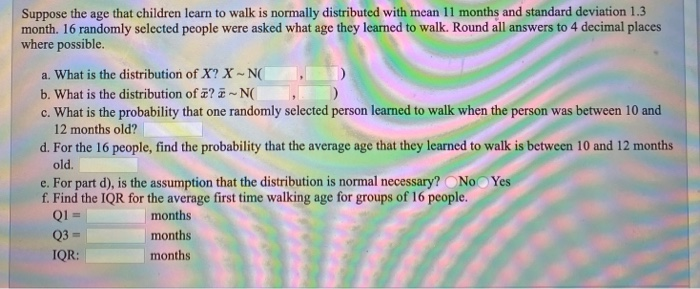Suppose the age that children learn to walk is normally distributed with mean 11 months and standard deviation 1.3 month. 16 randomly selected people were asked what age they learned to walk. Round all answers to 4 decimal places where possible. a. What is the distribution of X? X-NO b. What is the distribution of ? -NG c. What is the probability that one randomly selected person learned to walk when the person was between 10 and 12 months old?...

• ### A recent report in a women's magazine stated that the average age for women to marry in the United States is now 25 years of age, and that the standard deviation is assumed to be 3.2 years

A recent report in a women's magazine stated that the average age for women to marry in the United States is now 25 years of age, and that the standard deviation is assumed to be 3.2 years. A sample of 50 U.S. women is randomly selected. Find the probability that the sample mean age for women to marry is between 24 and 25 years. A: 0.4864 B: 0.6736 C: 0.9788 D: 0.1357

• ### Researchers report that children between the ages of 2 and 5 years watch an average of...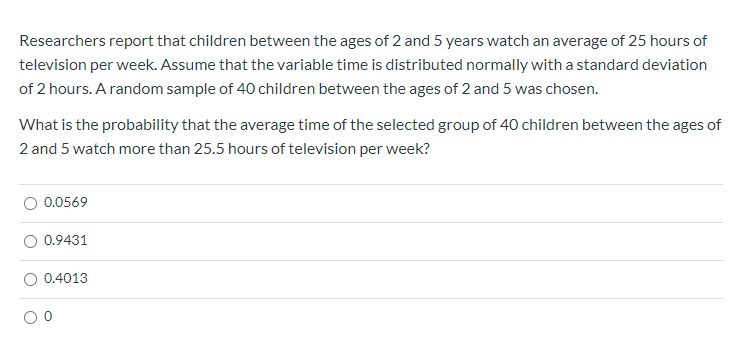Researchers report that children between the ages of 2 and 5 years watch an average of 25 hours of television per week. Assume that the variable time is distributed normally with a standard deviation of 2 hours. A random sample of 40 children between the ages of 2 and 5 was chosen. What is the probability that the average time of the selected group of 40 children between the ages of 2 and 5 watch more than 25.5 hours of...

• ### ne average age of doctors in a certain hospital is 43,0 years old. Suppose the distribution...ne average age of doctors in a certain hospital is 43,0 years old. Suppose the distribution of ages s normal and has a standard deviation of 8.0 years. If 25 doctors are chosen at random for a committee, find the probability that the average age of those doctors is less than 43.8 years. Assume that the variable is normally distributed [6 marks) 14. A mandatory competency test for high school students has a normal distribution with a mean of 400...